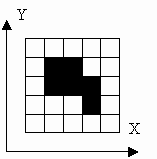## 1008. Image encoding

Time Limit: 2.0 second
Memory Limit: 16 MBThere are several ways to encode an image. In this problem we will consider two representations of an image. We assume that the image consists of black and white pixels. There is at least one black pixel and all black pixels are connected with their sides. Coordinates of black pixels are not less than 1 and not greater than 10. An example of such an image is at the figure.
Both representations describe arrangement of black pixels only.
At the first representation we specify in the first line number of black pixels and coordinates of each black pixel in the following lines. Pixels are listed in order of increasing X. In case of equality of X they are listed in order of increasing Y. Image at the figure is encoded as follows:
6
2 3
2 4
3 3
3 4
4 2
4 3
At the second representation we specify in the first line coordinates of the lowest left black pixel. Each of the following lines contains a description of neighbors for one of the pixels. At first, neighbors of the lowest left pixel are specified, then neighbors of its first neighbor (if it exists) are specified, then neighbors of its second neighbor (if it also exists) follow. When all its neighbors are described the description of the neighbors of its first neighbor follows. The description of the neighbors of its second neighbor follows then and so on.
Each descriptive line contains at most one letter for each neighbor: R for the right, T for the top, L for the left, B for the bottom. If the neighbor was already specified it is not included into the descriptive line and vice-versa. Also there is only one descriptive line for each pixel. Neighbors are listed counter-clockwise starting with the right. Each descriptive line except the last ends with a comma. The last line ends with a full stop. Image at the figure is encoded as follows:
2 3
RT,
RT,
,
B,
,
.
There are no leading or tailing spaces in any representation. There is exactly one space between X and Y coordinates.

### Input

One representation of the image will be given to your program in the input.

### Output

Your program has to write other representation of the image to the output.

### Sample

input output
```6
2 3
2 4
3 3
3 4
4 2
4 3
```
```2 3
RT,
RT,
,
B,
,
.
```
Problem Source: Third Open USTU Collegiate Programming Contest (PhysTech Cup), March 18, 2000

BFS：
 Accepted 0.015 217 KB

#include<iostream>
#include
<queue>
#include
<string.h>
#include
<string>
using  namespace std;

typedef
struct point
{
int x,y; } point;

bool graph={0};
bool f={0};
bool print={0};

int n;
int lbx=10,lby=10;

void input()
{

int x,y;

for(int i=1; i<=n; i++)
{
cin
>>x>>y; graph[x][y]=1;

if(x<lbx){ lbx=x; lby=y; }

else if(x==lbx&&y<lby)
lby
=y;
}
}

void BFS1()    //数字情况
{
queue
<point> q;
point temp; temp.x
=lbx; temp.y=lby;
cout
<<lbx<<' '<<lby<<endl;
q.push(temp);

int x,y; int cnt=0;
print[lbx][lby]
=1;

while(q.size())
{
temp
=q.front(); q.pop();
x
=temp.x; y=temp.y;

if(f[x][y]==1)continue;
f[x][y]
=1;

if(graph[x+1][y]==1&&!f[x+1][y])
{
if(!print[x+1][y]) cout<<'R'; print[x+1][y]=1; temp.x=x+1;temp.y=y; q.push(temp);}

if(graph[x][y+1]==1&&!f[x][y+1])
{
if(!print[x][y+1]) cout<<'T'; print[x][y+1]=1; temp.x=x;temp.y=y+1; q.push(temp);}

if(graph[x-1][y]==1&&!f[x-1][y])
{
if(!print[x-1][y]) cout<<'L'; print[x-1][y]=1; temp.x=x-1;temp.y=y; q.push(temp);}

if(graph[x][y-1]==1&&!f[x][y-1])
{
if(!print[x][y-1]) cout<<'B'; print[x][y-1]=1; temp.x=x;temp.y=y-1; q.push(temp);}

++cnt;

if(cnt==n) cout<<'.'<<endl;

else  cout <<','<<endl;
}
}

void BFS2()  // 从R T L B描述转换成坐标
{
lbx
=n; cin>>lby;
queue
<point>q;
point temp; temp.x
=lbx; temp.y=lby;
q.push(temp);

string str; int x,y;
graph[lbx][lby]
=1;

while(q.size())
{

temp
=q.front(); q.pop();
x
=temp.x; y=temp.y;

if(f[x][y])continue;
f[x][y]
=1;
cin
>>str;

for(int i=0; i<str.size()-1; i++)
{

if(str[i]=='R')     {graph[x+1][y]=1if(!f[x+1][y]){ temp.x=x+1; temp.y=y; q.push(temp);} }

else if(str[i]=='T'){graph[x][y+1]=1if(!f[x][y+1]){ temp.x=x; temp.y=y+1; q.push(temp);} }

else if(str[i]=='L'){graph[x-1][y]=1if(!f[x-1][y]){ temp.x=x-1; temp.y=y; q.push(temp);} }

else if(str[i]=='B'){graph[x][y-1]=1;if(!f[x][y-1]){ temp.x=x; temp.y=y-1; q.push(temp);} }
}

if(str[str.size()-1]=='.')break;
}

int cnt=0;

for(int i=1; i<=10; i++)

for(int j=1; j<=10; j++)

if(graph[i][j])cnt++;
cout
<<cnt<<endl;

for(int i=1; i<=10; i++)

for(int j=1; j<=10; j++)

if(graph[i][j])cout<<i<<' '<<j<<endl;
}

int main()
{
cin
>>n;

if(getchar()=='\n')
{
input();
BFS1();
}

else BFS2();
system(
"pause");

return 0;
}

posted on 2010-06-26 22:38 田兵 阅读(235) 评论(0)  编辑 收藏 引用 所属分类: URAL< 2010年6月 >
303112345
6789101112
13141516171819
20212223242526
27282930123
45678910

• 随笔 - 65
• 文章 - 2
• 评论 - 17
• 引用 - 0

•

• 积分 - 24167
• 排名 - 662